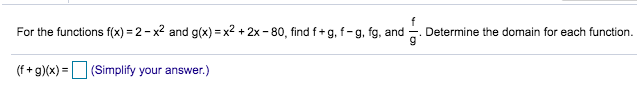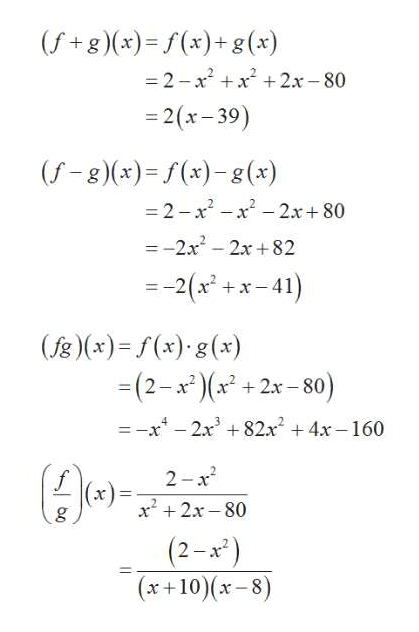# For the functions f(x) 2-x2 and g(x) x2 +2x -80, find f+ g, f- g, fg, andDetermine the domain for each function.(f+gx)(Simplify your answer.)=

Questionhelp_outlineImage TranscriptioncloseFor the functions f(x) 2-x2 and g(x) x2 +2x -80, find f+ g, f- g, fg, and Determine the domain for each function. (f+gx)(Simplify your answer.) = fullscreen
check_circleExpert Solution
Step 1

The given functions are f(x) = 2-x2 and g(x) = x2+2x-80

Step 2

The functions f+g, f-g, fg a...help_outlineImage Transcriptionclose(fg)(x)=f(x)+g (x) -2-x x + 2x-80 -2(x-39) (f-g)(x)=(x)-g (x) -2-x2 -x -2x+80 =-2x -2x+82 = 2(x x-41) + (fg)(x)f(x) g(x) (2-x22x-80) =-x -2x3 82x 4x-160 + 2 x x2x-80 (2-x) (x+10)(x-8) fullscreen

### Want to see the full answer?

See Solution

#### Want to see this answer and more?

Solutions are written by subject experts who are available 24/7. Questions are typically answered within 1 hour*

See Solution
*Response times may vary by subject and question
Tagged in

### Polynomials